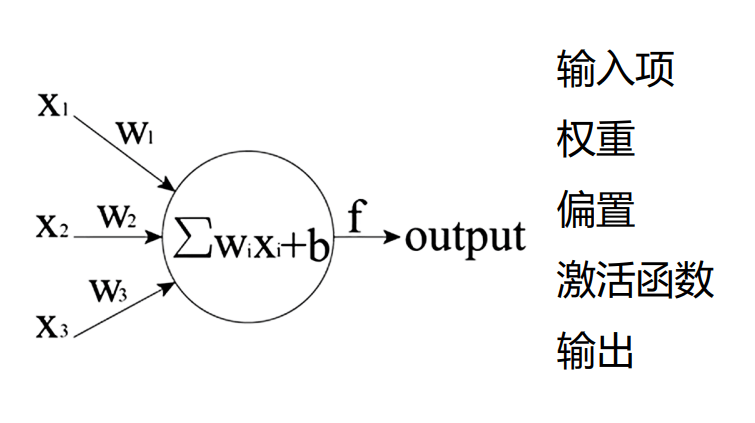# 初识BP神经网络(一)

## 神经元

• 输入项$X_i$
首先必须有输入
• 输入的权重$W_i$
表示这个输入的比重咋样，训练也是跳转这个比重
• 输入偏置b
为啥要加上这个偏置呢，其实是为了能整体上下移动，增加拟合能力
• 激活函数$f(x)$
就是防止数据太大而溢出的一个东西
• 输出
这个没啥好说的...## 反向传播

$f(X_1 * W_1 + b)$ = 0.3673

$0.3673 - 0.3=0.0673$

$f(x)=ax+b \\a=2,b=3,k=4\\st.期望输入x=1时候目标输出k=4$
$x=1$时，$f(x)$=5 我们要让输出更接近4咋办，我们让f(x)-4对a求偏导，算出a的梯度方向，也就是
$\frac{ \partial{ (f(x)-k)} }{ \partial a }=x$

$f(x)=ax+b \\a=2-1,b=3$

$x=1$$f(x)=4$，这不就一下子输出4了吗，此时这个迷你网络就训练好了！....吗？
$f(x)=ax+b \\a=1,b=3$

$b=b- \frac{ \partial{ (f(x)-4)} }{ \partial b }=b-1$

$f(x)=ax+b \\a=2,b=3$

$a=a- 0.1*\frac{ \partial{ (f(x)-k)} }{ \partial a }=a-0.1$
$b=b- 0.1*\frac{ \partial {(f(x)-k)} }{ \partial b }=b-0.1$
$f(x)=1.9x+2.9$
$x=1$$f(1)=4.8$

## 关于

NameSpace: bigonion.cn
Origin: bigonion.cn/blog